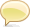# xdmp:describe

```xdmp:describe(
\$item as item()*,
[\$max-sequence-length as xs:unsignedInt?],
[\$max-item-length as xs:unsignedInt?]
) as xs:string```

### Summary

Returns a string representing the description of a given item sequence. If you take the output of this function and evaluate it as an XQuery program, it returns the item(s) input to the function.

Parameters
\$item The item sequence whose description is returned.
\$max-sequence-length Represents the maximum number of items per sequence to print. The default is 3. () means no maximum.
\$max-item-length Represents the maximum number of characters per item to print. The default is 64. The minimum is 8. () means no limit.

### Usage Notes

If you specify an item that is in a database, this function returns the path to the item (or to the items if you specify multiple items). If the item or items are constructed in XQuery, then it prints out the item, truncating the characters in each item according to the `\$max-item-length` parameter.

### Example

```xdmp:describe(current-date())

=> xs:date("2007-01-15-08:00")
```

### Example

```  let \$x := <mynode>Some text here.</mynode>
return
xdmp:describe(\$x)

=> <mynode>Some text here.</mynode>
```

### Example

```  (:  assume /mydoc.xml is an XML document with
the following content:
<mynode>Some text here.</mynode> :)
xdmp:describe(doc("/mydoc.xml")/mynode)

=> doc("/mydoc.xml")/mynode
```Stack Overflow: Get the most useful answers to questions from the MarkLogic community, or ask your own question.

## CommentsThe commenting feature on this page is enabled by a third party. Comments posted to this page are publicly visible.
• Check out Michael Blakeley excellent article on schemas which gives a good usage of xdmp:describe http://developer.marklogic.com/learn/2007-04-schema